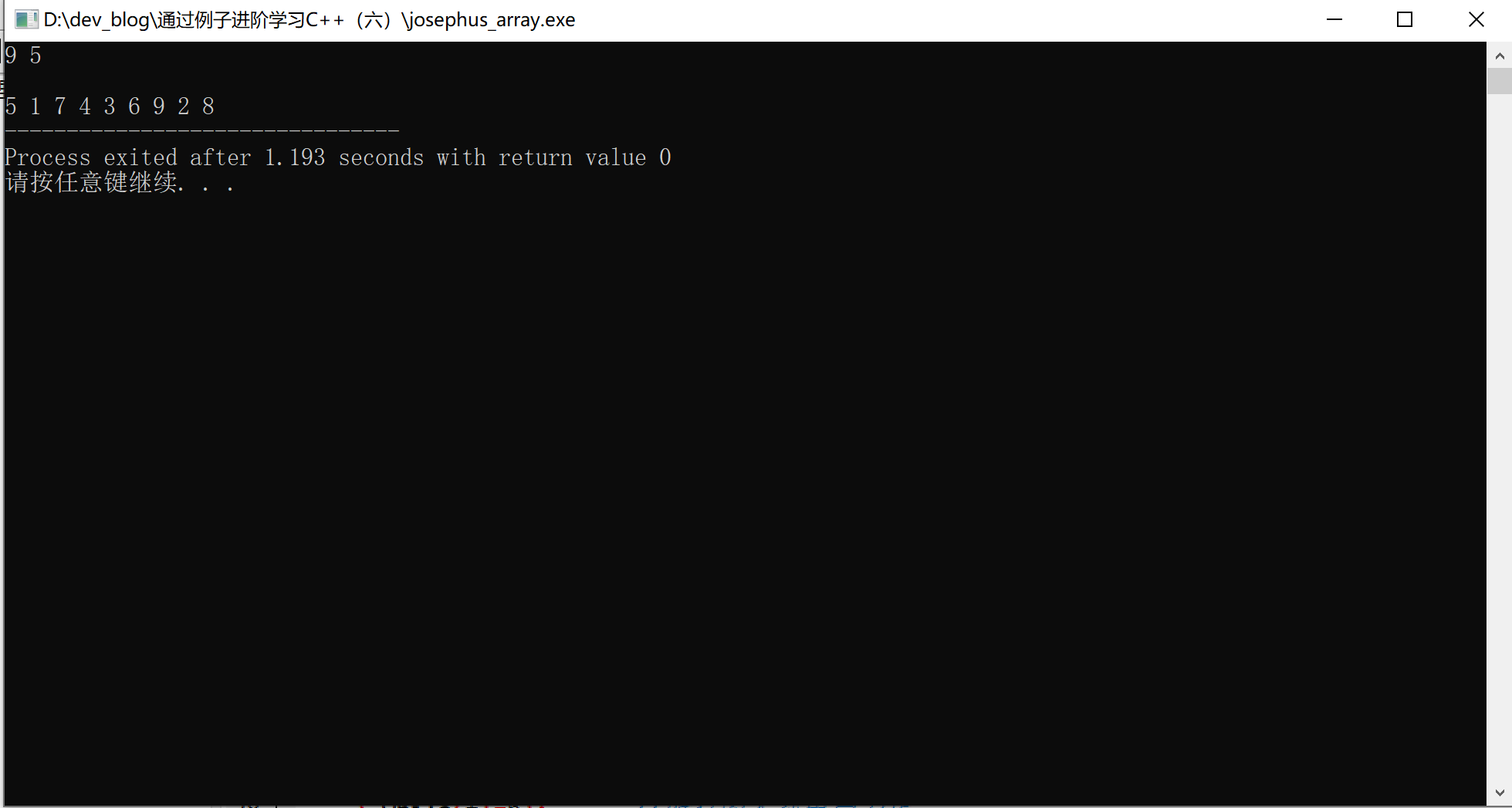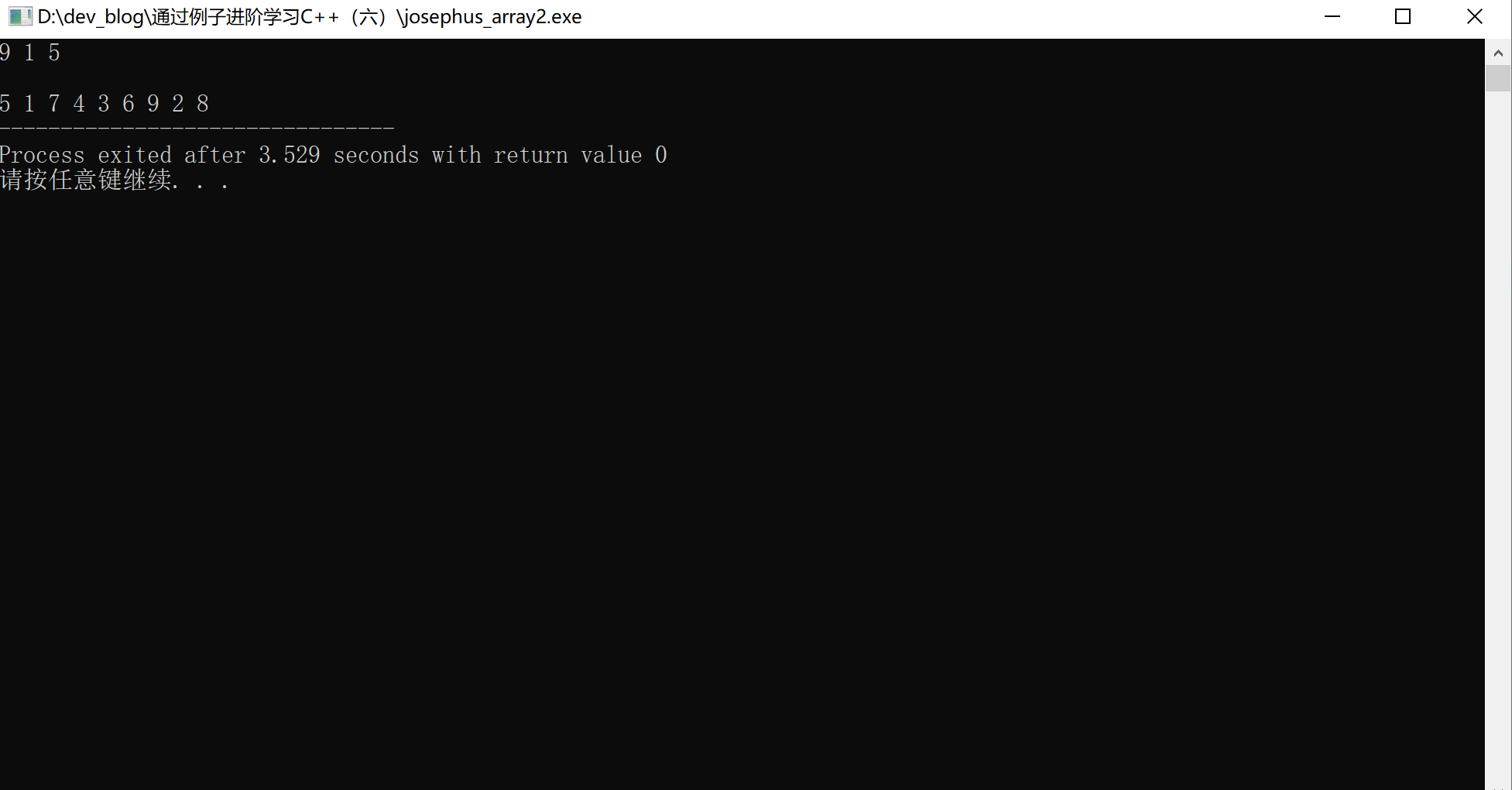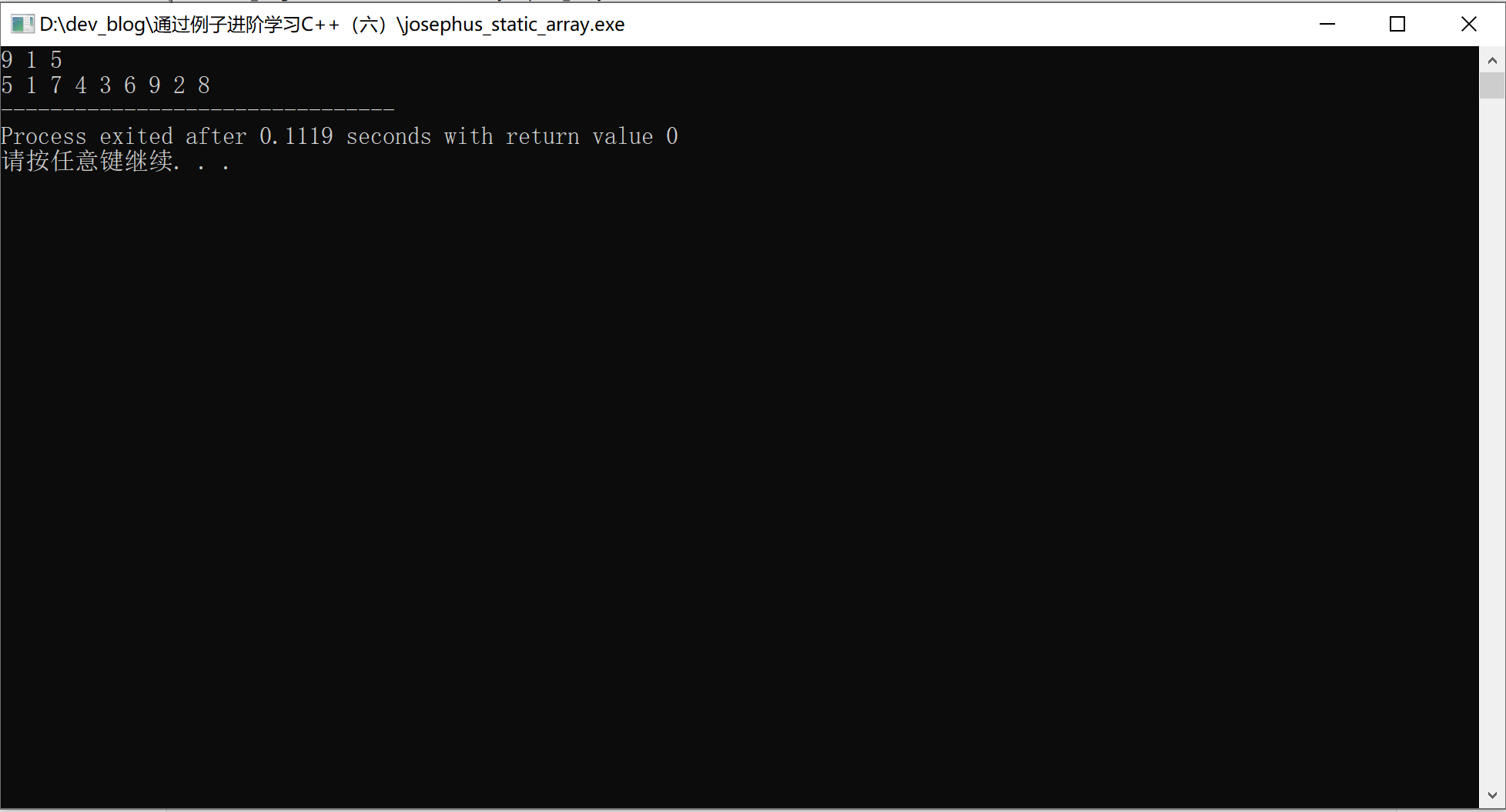# 1.问题描述

n 个人围坐在一个圆桌周围，现在从第 s 个人开始报数，数到第 **m **个人，让他出局；然后从出局的下一个人重新开始报数，数到第 m 个人，再让他出局......,如此反复直到所有人全部出局为止。

# 2.问题分析及用数组求解

​ 数组 bool a，可能会浪费了大量的存储空间；

​ 变量 t 从s=1开始数，指示当前数组的位置；

​ 变量 f 记录出局人数；

​ 变量 s 从1到m；

#include<iostream>
using namespace std;
int n,m,s,f,t;
bool a;
int main()
{
cin>>n>>m;		//共n人，从1开始数，数到m出局
for (int i=1;i<=n;++i){
a[i]=false;
}

t=0;//从数组a的a开始...记录数组a的第t个位置
f=0;//记录出局人数
s=0;//从1数到m，然后再从1数到m...
do
{
++t;
if (t==n+1) t=1;	//数到最后一个后，将t指向第一个
if (a[t]==false) ++s;	//第t个位置上有人则报数
if (s==m) 		//当前报的数是m
{
s=0;		//计数器清零
cout<<t<<" ";	//出局人的编号
a[t]=true;		//设置该位置已出局
f++;	 	//出局的人数加一
}
} while(f!=n);		//所有的人都出局为止
return 0;
}# 3.数组方式求解改进

#include<iostream>
using namespace std;
int n,m,s,f,t;
bool a;
int main()
{
cin>>n>>t>>m;		//共n人，从t开始数，数到m出局
cout<<endl;
for (int i=1;i<=n;++i){
a[i]=false;
}

t = t -1;
f=0;//记录出局人数
s=0;//从1数到m，然后再从1数到m...
do
{
++t;
if (t==n+1) t=1;	//数到最后一个后，将t指向第一个
if (a[t]==false) ++s;	//第t个位置上有人则报数
if (s==m) 		//当前报的数是m
{
s=0;		//计数器清零
cout<<t<<" ";	//出局人的编号
a[t]=true;		//设置该位置已出局
f++;	 	//出局的人数加一
}
} while(f!=n);		//所有的人都出局为止
return 0;
}# 4.静态链表实现约瑟夫环

#include<iostream>
using namespace std;

//约瑟夫环问题
void Josephus(int n,int s, int m)
{
cout<<n<<" "<<s<<" "<<m<<endl;
int i,j,k;
int *next= new int[n];
//初始化静态链表
for(i=0;i<n-1;++i){
next[i] = i+1;
}
next[n-1] = 0;

//k初始化为s的前一个位置,数组下标从0开始
if(s==1){
k = n-1;
}else{
k = s-2;
}

for(i=1;i<=n;++i){
//找到出局人的前驱
for(j=1;j<m;++j){
k=next[k];
}
cout<<next[k]+1<<" ";//数组下标从0开始，故需要+1
//数到m的人出列，删除该元素
next[k] = next[next[k]];
}
}
int main(){
Josephus(9,2,5);
return 0;
}# 5.总结

• 指针；
• 函数定义、调用；
• 通过优化求解约瑟夫环，加深问题的理解。

posted on 2020-01-17 09:38  siwei718  阅读(443)  评论(0编辑  收藏  举报